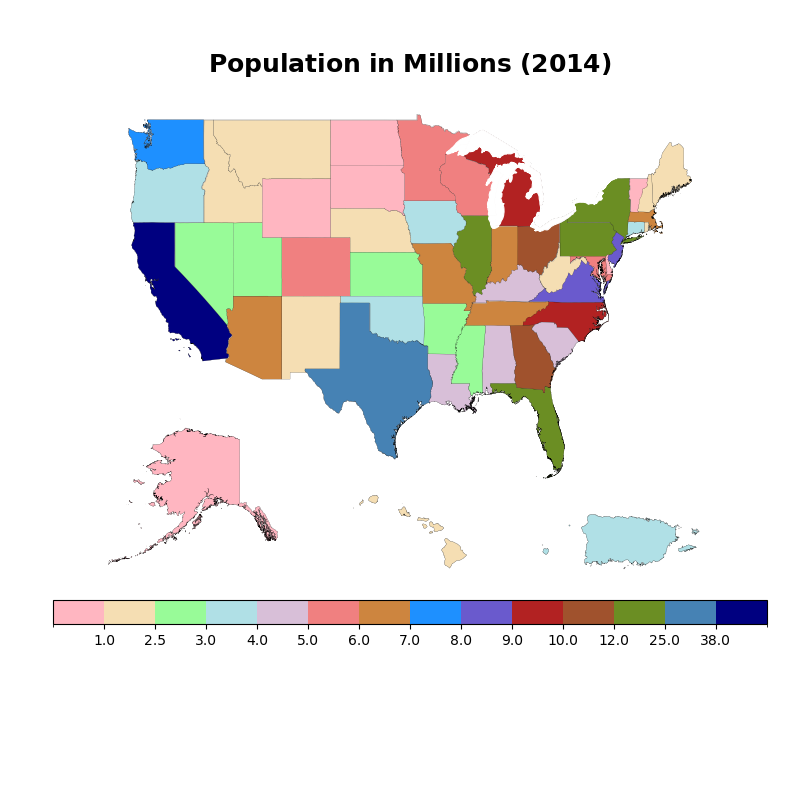# NCL_polyg_19.py#

This script illustrates the following concepts:
• Adding lines and polygons to a map

• Adding a map to another map as an annotation

• Coloring shapefile outlines based on an array of values

• Drawing a custom colorbar on a map

• Using functions for cleaner code

• Overlaying a shape from one shapefile over another

See following URLs to see the reproduced NCL plot & script:

Import packages:

import matplotlib.pyplot as plt
import matplotlib.gridspec as gridspec
from matplotlib.collections import PatchCollection
from matplotlib.patches import Polygon
import matplotlib.colors as colors
from mpl_toolkits.axes_grid1.inset_locator import inset_axes
import matplotlib.cm as cm
import shapefile as shp
import numpy as np

import geocat.datafiles as gdf
import geocat.viz as gv


# Open all shapefiles and associated .dbf, .shp, and .prj files so sphinx can run and generate documents

# Open the text file with the population data
state_population_file = open(gdf.get("ascii_files/us_state_population.txt"),
'r')
# Open shapefiles


Set colormap data and colormap bounds:

colormap = colors.ListedColormap([
'lightpink', 'wheat', 'palegreen', 'powderblue', 'thistle', 'lightcoral',
'peru', 'dodgerblue', 'slateblue', 'firebrick', 'sienna', 'olivedrab',
'steelblue', 'navy'
])

colorbounds = [0, 1, 2.5, 3, 4, 5, 6, 7, 8, 9, 10, 12, 25, 38, 40]

norm = colors.BoundaryNorm(colorbounds, colormap.N)


Define helper function to get the populations of each state

def getStatePopulations(state_population_file):

population_dict = {}
for line in Lines:
nameandpop = line.split(" ")
if nameandpop[-1].isnumeric():
name = nameandpop
pop = (int)(nameandpop[-1]) / 1000000
population_dict[name] = pop
return population_dict


Define helper function to get the color of each state based on its population

def findDivColor(colorbounds, pdata):

for x in range(len(colorbounds)):

if pdata >= colorbounds[len(colorbounds) - 1]:
return colormap.colors[x - 1]
if pdata >= colorbounds[x]:
continue
else:
# Index is 'x-1' because colorbounds is one item longer than colormap
return colormap.colors[x - 1]


Define helper function to remove ticks from axes

def removeTicks(axis):

axis.get_xaxis().set_visible(False)
axis.get_yaxis().set_visible(False)


Define helper function to plot and color each state

def plotRegion(region, axis, xlim, puertoRico, waterBody):

# Create empty lists for filled polygons or" patches" and "water_patches"
patches = []
water_patches = []

# Plot each shape within a region (ex. mainland Alaska and all of it's surrounding Alaskan islands)
for i in range(len(region.shape.parts)):

i_start = region.shape.parts[i]
if i == len(region.shape.parts) - 1:
i_end = len(region.shape.points)
else:
i_end = region.shape.parts[i + 1]

# Create new empty lists to hold lat and lon coordinates
x = []
y = []

# Get every coordinate within every shape (as long as it is within the x coordinate limits)
for i in region.shape.points[i_start:i_end]:
if xlim is not None and i < xlim:
continue
if xlim is not None and i > xlim:
continue
else:
x.append(i)
y.append(i)

# Plot outline of each region
axis.plot(x, y, color='black', linewidth=0.1, zorder=1)

# Fill each state with color:
# Determine the region to be plotted (Puerto Rico or United States)
if waterBody is False:
if puertoRico is False:
abbrevname = shape.record[-1].split(".")
abbrevstate = abbrevname
else:
abbrevstate = 'PR'

# If the region being plotted is a state with a population
if waterBody is False:
pop = population_dict[abbrevstate]
color = findDivColor(colorbounds, pop)
# Set characteristics and measurements of each filled polygon "patch"
patches.append(
Polygon(np.vstack((x, y)).T, True, color=color, linewidth=0.1))

# If the region being plotted is a body of water with no population
else:
# Set characteristics and measurements of each filled polygon "patch"
water_patches.append(
Polygon(np.vstack((x, y)).T, True, color='white', linewidth=.7))

pc = PatchCollection(patches,
match_original=True,
edgecolor='k',
linewidths=0.1,
zorder=2)
# Plot filled region on axis

wpc = PatchCollection(water_patches,
match_original=True,
edgecolor='white',
linewidth=.8,
zorder=3)
# Plot filled region on axis


Plot:

# Create figure
fig = plt.figure(figsize=(8, 8))
spec = gridspec.GridSpec(ncols=1,
nrows=2,
hspace=0.05,
wspace=0.1,
figure=fig,
height_ratios=[2, 1])

# Create upper axis
removeTicks(ax1)

# Create lower axis
removeTicks(ax2)

# Create three inset axes on lower axis for Alaska, Hawaii, and Puerto Rico respectively
axin1 = ax2.inset_axes([0.0, 0.7, 0.30, 0.80], frameon=False)
removeTicks(axin1)
axin2 = ax2.inset_axes([0.40, 0.7, 0.20, 0.40], frameon=False)
removeTicks(axin2)
axin3 = ax2.inset_axes([0.70, 0.7, 0.30, 0.30], frameon=False)
removeTicks(axin3)

# Get population of each state
population_dict = getStatePopulations(state_population_file)

# Plot every shape in the US shapefile
for shape in us.shapeRecords():

plotRegion(shape, axin1, [None, 100], puertoRico=False, waterBody=False)
elif shape.record == 'Hawaii':
plotRegion(shape,
axin2, [-161, None],
puertoRico=False,
waterBody=False)
else:
plotRegion(shape, ax1, [None, None], puertoRico=False, waterBody=False)

# Plot every shape in the puerto rico shapefile
for shape in pr.shapeRecords():
plotRegion(shape, axin3, [None, None], puertoRico=True, waterBody=False)

# Plot every body of water shape in the detailed US shapefile
for shape in usdetailed.shapeRecords():
if shape.record == 'Water body':
plotRegion(shape, ax1, [None, None], puertoRico=False, waterBody=True)

# Set title using helper function from geocat-viz
title = r"$\bf{Population}$" + " " + r"$\bf{in}$" + " " + r"$\bf{Millions}$" + " " + r"$\bf{(2014)}$"
gv.set_titles_and_labels(ax1, maintitle=title, maintitlefontsize=18)

# Create fourth inset axis for colorbar
axin4 = inset_axes(ax2, width="115%", height="12%", loc='center')

# Create colorbar
cb = fig.colorbar(cm.ScalarMappable(cmap=colormap, norm=norm),
cax=axin4,
boundaries=colorbounds,
ticks=colorbounds[1:-1],
spacing='uniform',
orientation='horizontal')

plt.show()/home/docs/checkouts/readthedocs.org/user_builds/geocat-examples/checkouts/latest/Gallery/Polygons/NCL_polyg_19.py:167: MatplotlibDeprecationWarning: Passing the closed parameter of __init__() positionally is deprecated since Matplotlib 3.6; the parameter will become keyword-only two minor releases later.
Polygon(np.vstack((x, y)).T, True, color=color, linewidth=0.1))
/home/docs/checkouts/readthedocs.org/user_builds/geocat-examples/checkouts/latest/Gallery/Polygons/NCL_polyg_19.py:173: MatplotlibDeprecationWarning: Passing the closed parameter of __init__() positionally is deprecated since Matplotlib 3.6; the parameter will become keyword-only two minor releases later.
Polygon(np.vstack((x, y)).T, True, color='white', linewidth=.7))


Total running time of the script: ( 0 minutes 21.870 seconds)

Gallery generated by Sphinx-Gallery